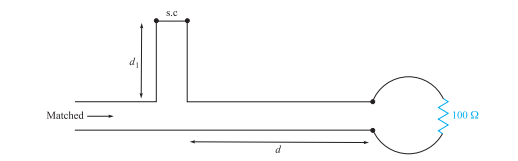### Create an Account

Home / Questions / Repeat Problem 1037 with Z0 50Ω and RL Rg 25 Ω Carry out the analysis for the time peri...

# Repeat Problem 1037 with Z0 50Ω and RL Rg 25 Ω Carry out the analysis for the time period 0 Problem In the transmission line of Figure

Repeat Problem 10.37, with Z0 = 50Ω, and RL = Rg = 25 Ω. Carry out the analysis for the time period 0

Problem

In the transmission line of Figure 10.20, Rg = Z0 = 50 φ, and RL = 25 Ω. Determine and plot the voltage at the load resistor and the current in the battery as functions of time by constructing appropriate voltage and current reflection diagramsJun 13 2020 View more View LessSubscribe To Get Solution